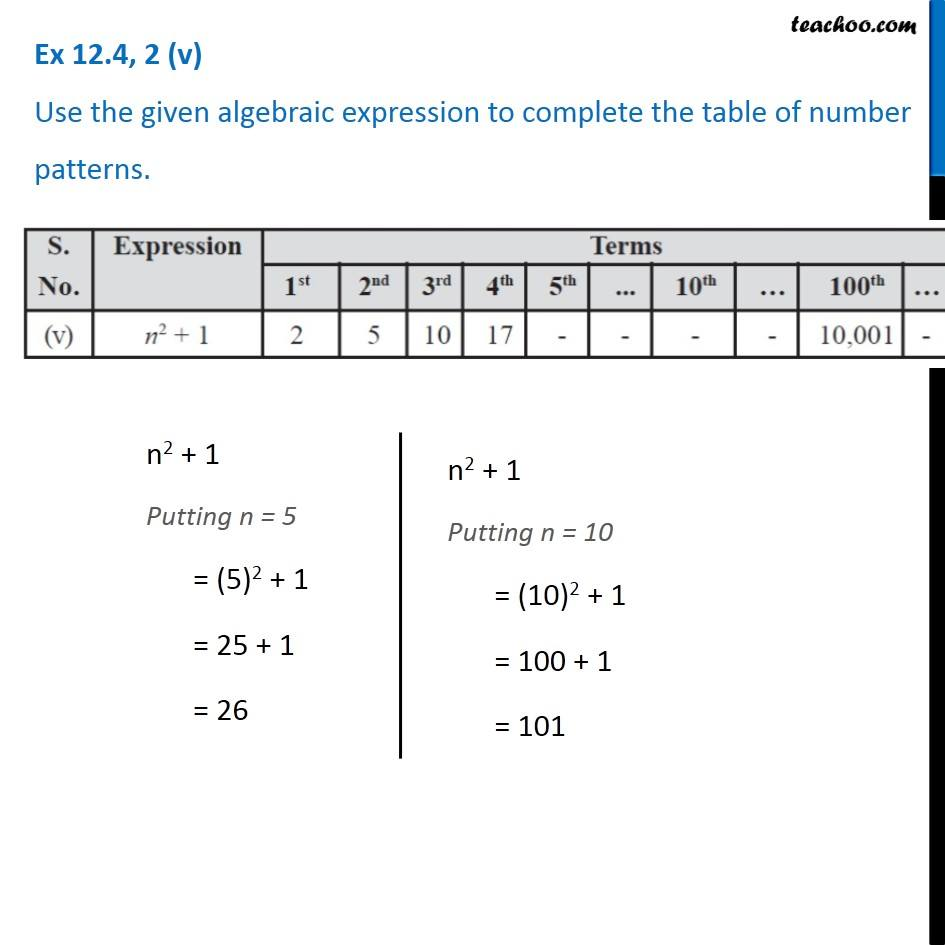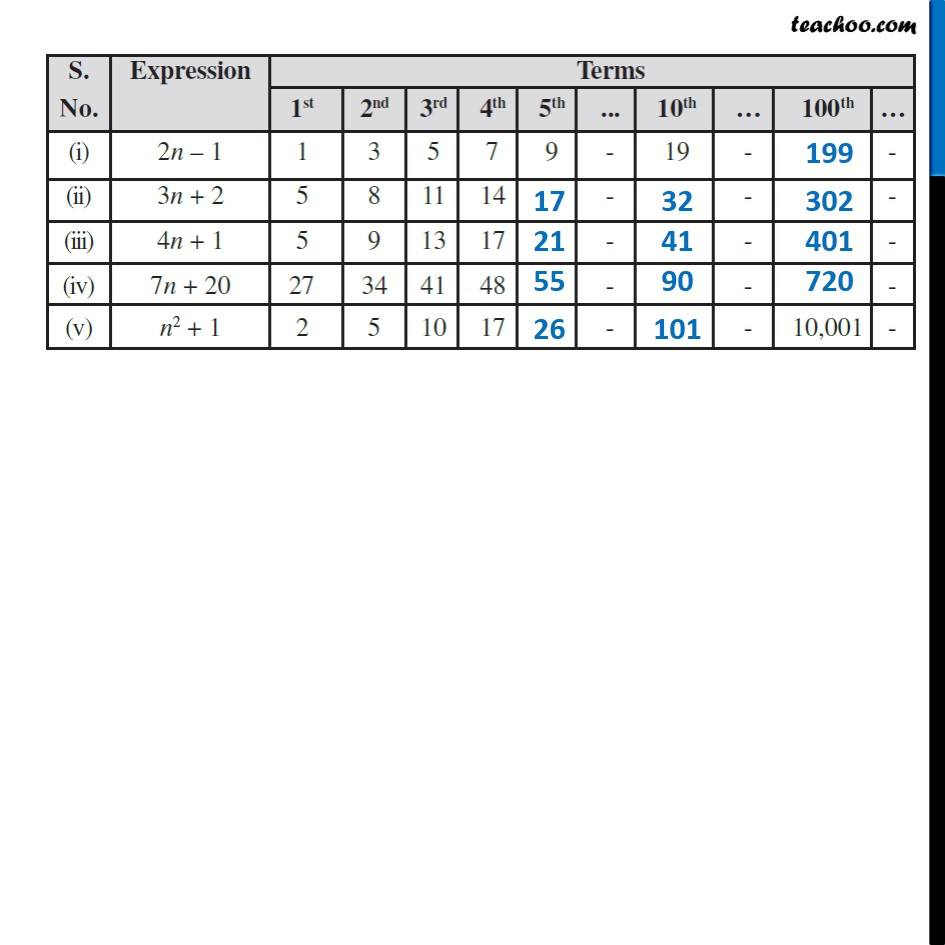Observing patterns

Chapter 10 Class 7 Algebraic Expressions
Serial order wiseLearn in your speed, with individual attention - Teachoo Maths 1-on-1 Class

### Transcript

Question 2 (v) Use the given algebraic expression to complete the table of number patterns. n2 + 1 Putting n = 5 = (5)2 + 1 = 25 + 1 = 26 n2 + 1 Putting n = 10 = (10)2 + 1 = 100 + 1 = 101